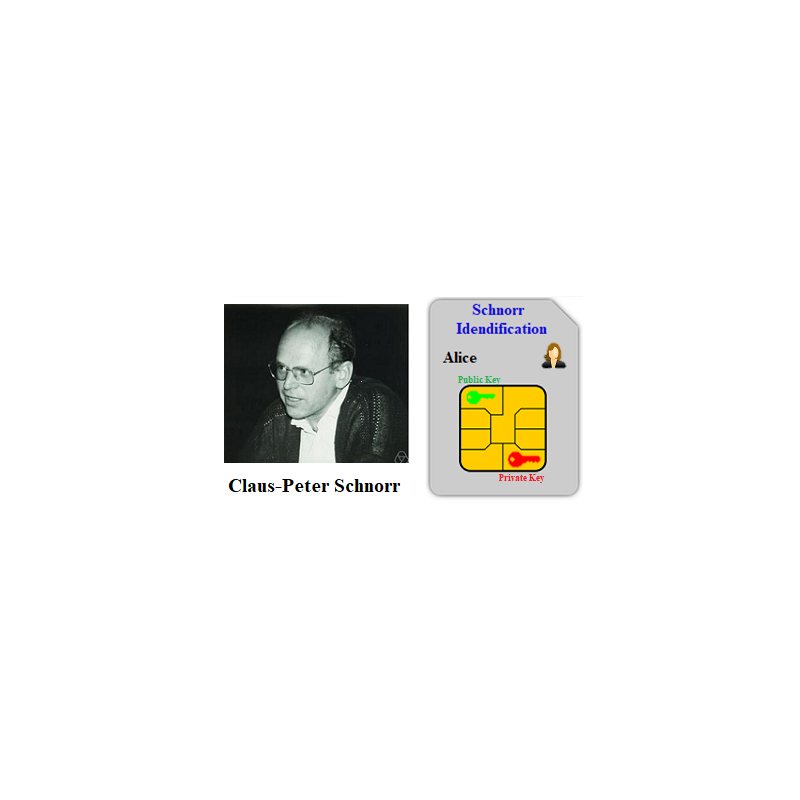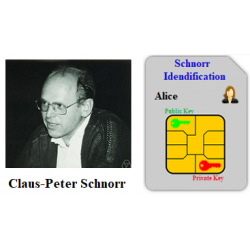•# Schnorr Identification

€3.00
Tax included

Schnorr Identification scheme.

Quantity

This protocol is executed between Student Alice (You) and Mentor Bob. You must self-sign your Public key A=gx mod p computed for the Schnorr identification algorithm and send it to the Mentor for verification. Mentor replies You with his self-signed Public Key. Both signatures are created using Schnorr algorithm. You must verify Mentor’s signature to be aware that he is the same person that carried out Schnorr identification protocol.

System parameters for this protocol are the same as in Schnorr identification protocol and are the same for all students. They have the following values: p=157718819 and g=8, where p is a strong prime. Please check it for awareness.

Mentor Bob Public Key is B=80094402 generated using these system parameters.

Parameter values sent by Alice are included in brackets [ ] and must be entered in the corresponding input fields.

The following functions are used in the protocol:

>> mod_exp(g,x,p)

>> randi(z)

>> H28(“D”)

1.      Find your previously saved Private and Public Keys and send [A] to the Mentor.

2.      Mentor signed his Public Key B=80094402 generated using system parameters p=157718819  and g=8 and obtaining a signature S=(w, r), where w=gk mod p=….. and r=k-yh mod (p-1)=….. . Recall that k is a random number, y is Mentor’s Private Key and h is a H-function of Mentor’s whitness concatenated with his Public Key, i.e. h=H28(w||B). Compute h and verification parameter v=gr∙ah mod p. Decide if the Mentor‘s signature is valid and send your decision Yes/No and computed value v, i.e. [Y/N, v] to the Mentor.

3.       If it is OK, Mentor asks you to sign your Public Key in the same way as in step 2. Compute your signature S1=(w1, r1) and send [w1, r1] to the Mentor for verification.

If it is not OK, follow the Mentor’s advise.

3379 Items